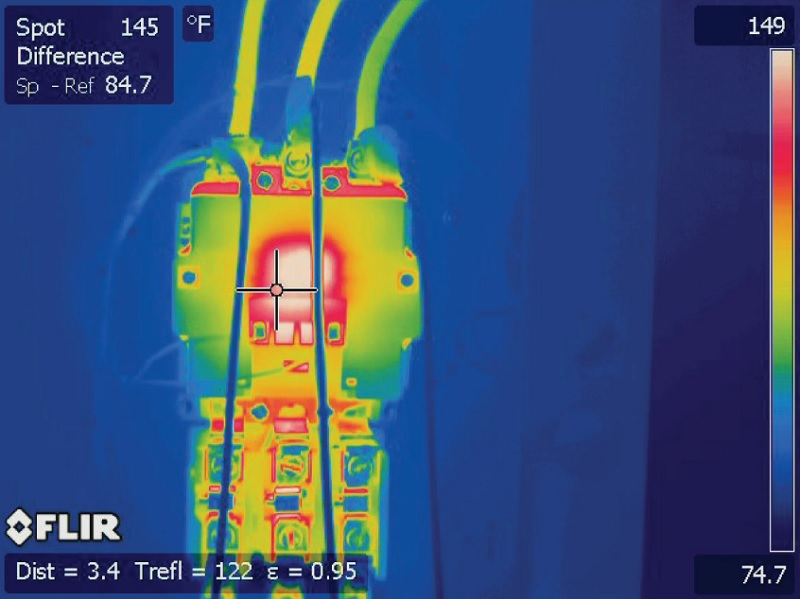# Temperature Unit Conversion Calculator

## Conversion between temperature units is perhaps one of the most common simple linear formula applications. This calculator provides easy conversion and explains the reason for the odd Fahrenheit units.

### Temperature

Temperature is a measurement of the kinetic energy contained in the molecules of matter in an object. Solids, liquids, and gasses will all have some sort of temperature as long as there is any kinetic activity.

#### Absolute Zero Temperature

The only instance in which no kinetic energy is present would be under the subject of ‘absolute zero’ temperature, which is currently only a hypothetical scenario. Only one unit system considers this ‘zero’ energy to be equal to ‘zero’ degrees, and this is the Kelvin scale. To achieve this absolute zero temperature, one would have to start at the freezing point, then fall another 273 degrees Celsius (523 degrees Fahrenheit)!#### Temperature Conversion Formulas

One degree in the Celsius scale is equal to one Kelvin (formally stated without the word ‘degrees’), so the conversion from degrees Celsius to Kelvins is simply:

$$^\circ Celsius+273.15=Kelvins$$

Conversion from Celsius to Fahrenheit is more complicated, since the degrees are a different magnitude, and the freezing point is non-zero in the Fahrenheit scale. This leads to a linear equation in slope-intercept form:

$$^\circ Fahrenheit =~ ^\circ Celsius \times \frac{9}{5}+32$$

#### Why Does Water Boil at 212 Fahrenheit?

A common question regarding temperature scales is the rationale for Fahrenheit and the seemingly arbitrary values of 32 and 212 degrees for freezing and boiling, respectively.

According to Daniel Fahrenheit, the founder of the scale, a standard of freezing was, in fact, 0 degrees. However, his standard process involved recording the freezing point of a salty brine mixture (similar to seawater) which freezes at a lower temperature than pure water.

In this scale, a second reference point was chosen to be the temperature of the human body, which Fahrenheit chose to be 90 degrees, and by using this scale, it appeared that pure water would freeze at 30 degrees. This is consistent (both in number and by name) with geometric degrees forming angular positions.

In order to more easily create accurate scales, the temperature values of freezing and body temperature were shifted to 32 and 96 degrees, with 64 degrees separating the two values. This number can be divided by two quite easily many times, as any binary logic enthusiast can tell you.

With this scale, the boiling point of water was found to be about 212 degrees, and a standard we set in place to establish these values at exactly 32 and 212 degrees, with exactly 180 degrees between—once again maintaining the familiar geometric relationship.

Related Content:

Textbook

Articles